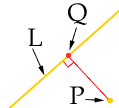Name: Kat Who is asking: Student Level: Secondary Question: Maybe one of you guys can help me with this, I'm really stuck. Find the shortest distance from the given line. Round to the nearest tenth. (-2,-1) and x+5y+20=0 Thanks a bunxh! Hi Kat, What you need to realize is that: the shortest distance from a point P to a line L is the perpendicular distance. and if a line has slope m then any line perpendicular to it has slope -1/m To solve your problem you can proceed as follows: Solve the equation of line L: x + 5y + 20 = 0 for y to get an equation of the form y = mx + b. m is then the slope of L. Write the equatiion of the line through P = (-2,-1) with slope -1/m. (This is the red line in the diagram.)Find the point Q where these two line intersect. Find the distance from P to Q. I hope this helps, Penny Go to Math Central